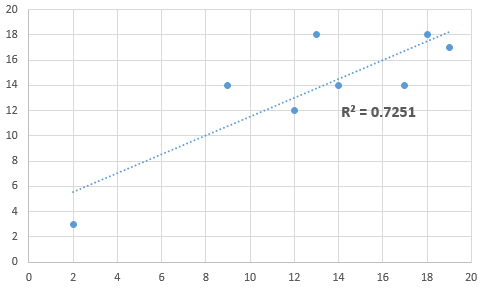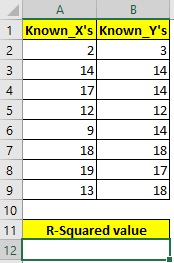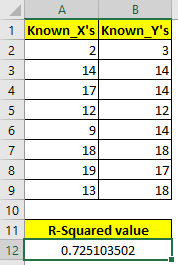# How to Find R-Squared in Excel? Use (RSQ) Function

What is r squared in excel?

The R-Squired of a data set tells how well a data fits the regression line. It is used to tell the goodness of fit of data point on regression line. It is the squared value of correlation coefficient. It is also called co-efficient of determination.  This is often used in regression analysis, ANOVA etc. analysis. The squired formula in excel is RSQ function. In this tutorial, we will learn how to calculate r squared in excel using RSQ function.How to calculate R square in excel?

As I told you, Excel provides RSQ function to easily get the R-squired of the sample data set.

Syntax:

 =RSQ(Known_ys, Known_xs)

Known_ys: The dependent variables.

Known_xs: The independent variables.

Note: The number of variables of x and y should be the same. Otherwise excel will through #NA error.

Now, let's have an example of RSQ function so that we can understand it.

Example of R-Square in Excel

Here I have a sample data set. In range A2:A9, I have know_xs and in range B2:B9, I have known_ys.Now, let us use the RSQ function to find R2 in excel. Write this formula in cell A12.

 =RSQ(B2:B9,A2:A9)When you hit the enter button you get the value 0.725103502, which is 72% approx.

How to Interpret R-Squared

The R-Squared value always falls in the range 0.0-1.0 or we can say 0% to 100%. 0% r-squared value tells that there is no guarantee of falling a data point on the regression line. Where 100% r-squared value tells us that there are 100% chances of falling data point on regression line. (There are other factors and analysis too that are done to assure this.

In our case, there 72% of goodness of fit.

Another method of Calculating R-Squared in Excel

In the beginning of the article, I told you that R-squared value is squared value of correlation coefficient. So if you write this formula, it will return the same result as RSQ function:

 =POWER(CORREL(A2:A9,B2:B9),2)

Here, CORREL function is used to calculate correlation coefficient and then encapsulated it with POWER function to get the square of the correlation coefficient.

I hope it was explanatory enough. To understand r-square more, read regression analysis in excel. For further queries use the comments  section below.

Terms and Conditions of use

The applications/code on this site are distributed as is and without warranties or liability. In no event shall the owner of the copyrights, or the authors of the applications/code be liable for any loss of profit, any problems or any damage resulting from the use or evaluation of the applications/code.